• LSTM 时间序列分析预测 目录 使用LSTM神经网络进行时间序列数据预测分析。 基于Tensorflow框架、Kerase接口开发网络模型。 包含数据清洗，数据特征提取，数据建模，数据预测。 想学习更多深度学习项目，可访问如下...
• 使用LSTM构建聊天机器人，无论输入是什么，就算输入完全不符合语法，模型都会给出一个输出，显然，这个输出不是我们想要的，如何识别模型输出是不是我们想要的？我们需要一种评估指标，评估模型输出的置信度。那么...
       使用LSTM构建聊天机器人，无论输入是什么，就算输入完全不符合语法，模型都会给出一个输出，显然，这个输出不是我们想要的，如何识别模型输出是不是我们想要的？我们需要一种评估指标，评估模型输出的置信度。那么使用loss值做为评价指标是否可行呢？具体的想法是输入一句话（string）进LSTM模型，得到预测句子，把预测句子做为标签（label），即（string，label）做为一条对话，输入到LSTM训练模型，计算出loss值loss_val，loss_val除以模型训练时loss平均值做为LSTM模型的置信度指标。这个想法是否可行，写代码测试看看。从下图测试结果来看，训练loss值：最小0.0，最大0.000322，平均2.495e-6；语料集之内的对话测试3次loss值：6.557e-7，0.0，0.219；语料集之外的对话测试3次loss值：2.187e-5，0.0，0.0886，语料集之内与之外的loss值并没与明显的边界，因此不能做为LSTM模型的置信度指标。

import sys
import pickle
import time

import numpy as np
import tensorflow as tf
from flask import Flask,request
import re

import relavance
from sequence_to_sequence import SequenceToSequence
from data_utils import batch_flow

def test(params):

x_data, _ = pickle.load(open('pkl/chatbot.pkl', 'rb'))
ws_encode = pickle.load(open('pkl/ws_encode.pkl', 'rb'))
ws_decode = pickle.load(open('pkl/ws_decode.pkl', 'rb'))
relv = pickle.load(open('pkl/relevance.pkl','rb'))

for x in x_data[:5]:
print(' '.join(x))

config = tf.ConfigProto(
device_count = {'CPU':1, 'GPU':0},
allow_soft_placement=True,
log_device_placement=False
)

save_path = './model/s2ss_chatbot_anti.ckpt'

#tf.reset_default_graph()
graph_pred = tf.Graph()
graph_loss = tf.Graph()

with graph_pred.as_default():
model_pred = SequenceToSequence(
input_vocab_size=len(ws_encode),
target_vocab_size=len(ws_decode),
batch_size=1,
mode='decode',
beam_width=0,
max_decode_step=2,
**params
)
init_pred = tf.global_variables_initializer()

with graph_loss.as_default():
model_loss = SequenceToSequence(
input_vocab_size=len(ws_encode),
target_vocab_size=len(ws_decode),
batch_size=1,
**params
)
init_loss = tf.global_variables_initializer()

sess_loss = tf.Session(graph=graph_loss,config=config)
sess_loss.run(init_loss)

with tf.Session(graph=graph_pred,config=config) as sess:
sess.run(init_pred)
model_pred.load(sess, save_path)
model_loss.load(sess_loss,save_path)

while True:
user_text = input('请输入您的句子:')
if len(user_text)<1 or user_text in ('exit', 'quit'):
sess_loss.close()
print("test exit.")
exit(0)

x_test = [list(user_text.lower())]
bar = batch_flow([x_test], ws_encode, 1)
x, xl = next(bar)
x = np.flip(x, axis=1)

pred = model_pred.predict(sess,np.array(x),np.array(xl),
attention=False,ws = None)

loss = model_loss.train(sess_loss,np.array(x),np.array(xl),
pred,np.array([len(pred)]), loss_only=True)
print("loss:", loss)

ask = ''.join(ws_encode.inverse_transform(x)[::-1])

print("input:",ask)

for p in pred:
if len(p) > 0:
ans = ws_decode.inverse_transform(p)

print("answer:", ''.join(ans))
print("\n")

def main():
import json

time.sleep(1)
print("start test...")
test(json.load(open('params.json')))

if __name__ == '__main__':
main()


训练时loss值：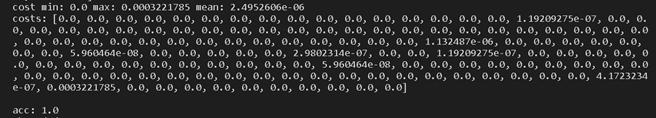语料集之内的对话测试结果：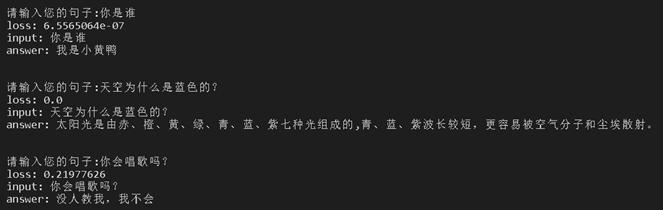语料集之外的对话测试结果：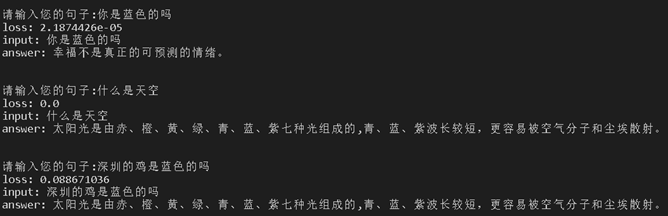展开全文NLP 人工智能
• MinMaxScaler计算数据集的汇总统计量，并产生一个MinMaxScalerModel。注意因为零转换后可能变为非零，所以即便为稀疏输入，输出也可能为稠密向量。 该模型可以将独立的特征的转换到指定的范围内
LSTM用于股票预测

库

使用到的库：Pandas库

一，数据处理

一，加载数据集

二，获取第六列数据，转成列表

三，对每一列数据做归一化处理.（MinMaxScaler）

MinMaxScaler作用是每一列，即每一维特征。将每一维特征线性地映射到指定的区间，通常是[0, 1]。MinMaxScaler计算数据集的汇总统计量，并产生一个MinMaxScalerModel。注意因为零值转换后可能变为非零值，所以即便为稀疏输入，输出也可能为稠密向量。
该模型可以将独立的特征的值转换到指定的范围内。
它也有两个参数可以设置：

min: 默认为0。指定区间的下限。
max: 默认为1。指定区间的上限。
二，LSTM网络

# create and fit the LSTM network, optimizer=adam, 25 neurons, dropout 0.1
# 创建并适应LSTM网络，优化器=adam, 25个神经元，dropout 0.1
model = Sequential()
model.add(LSTM(25, input_shape=(1, look_back)))
model.add(Dropout(0.1)) #损失值：0.1
model.add(Dense(1)) #全连接层，以(*,1)数组输出
model.compile(loss='mse', optimizer='adam') #用于配置训练模型 optimizer：优化器adam，loss:mse
model.fit(trainX, trainY, epochs=1000, batch_size=240, verbose=1)

功能显示：

为什么没有显示预测多少，它就预测了未来这么多的数量？

1.数据的变化

数据归一化处理：（5024，1）

数据分训练集和测试集：（2517，1），（2517，1）

构造新的数据，数组转成矩阵（二维张量）（为什么不要最后的240数据，倒数的前2276数据包含最后的数据240.）：（2276，240）

将二维张量构造为三维张量，将输入重塑为[样本、时间步长、特征]：(2276, 1, 240)

待输入模型的数据，trainX.shape前2276，trainY.shape从240到2516的2276.：trainX.shape，(2276, 1, 240)；trainY.shape，(2276,).

2.程序的结构图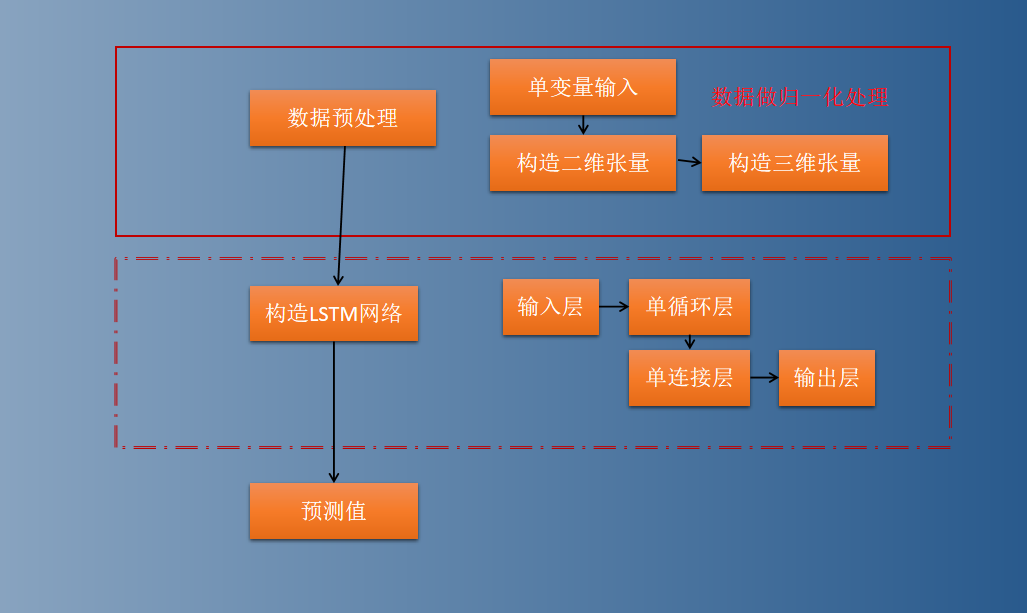3改变参数，epochs：训练模型迭代的次数为1000，2000，10000的时候.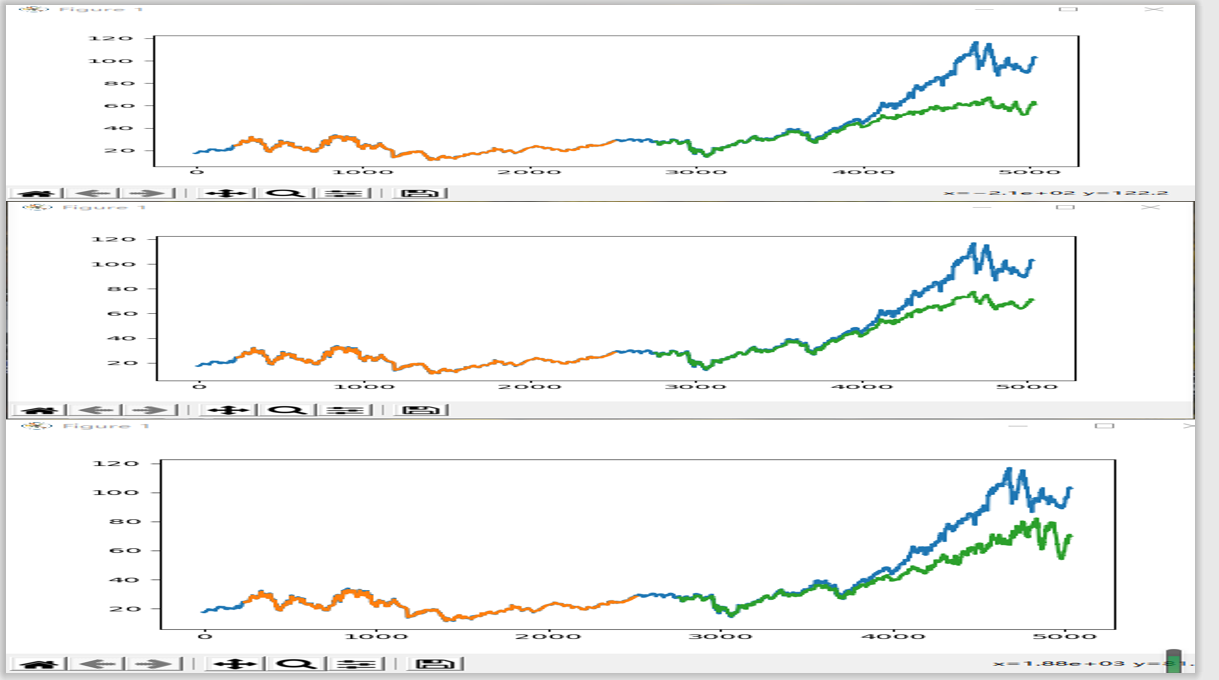4.基于LSTM的股票收盘价预测分析


展开全文• ## LSTM

2020-08-09 13:39:10
h，对短期的输入十分敏感，但是对于长期的输入，就不能很好的记忆其信息，LSTM 设计了 3 个门，输入门、遗忘门和输出门，不仅学习在 t 时刻的隐藏状态 hhh，还会多学习一个 ccc 来形成长期的记忆，使得 LSTM 可以...
LSTM
长短期记忆网络，适合于处理和预测时间序列中间隔和延迟相对较长的重要事件，LSTM 是为了解决 RNN 结构中存在的”梯度消失“的问题而提出的。RNN 只有一个隐状态 h，对短期的输入十分敏感，但是对于长期的输入，就不能很好的记忆其信息，LSTM 设计了 3 个门，输入门、遗忘门和输出门，不仅学习在 t 时刻的隐藏状态 $h$，还会多学习一个 $c$ 来形成长期的记忆，使得 LSTM 可以记住长期的信息
LSTM 的输入与输出
在 t 时刻，LSTM 有 三个输入

当前网络的输入值：$x_t$
上一时刻 LSTM 的输出值： $h_{t-1}$
上一时刻的单元状态：$c_{t-1}$

t 时刻，有两个输出

t 时刻的隐藏状态$h_t$
t 时刻的单元状态$c_t$

门的概念
如果门的输出是 0，就表示门关闭，如果门的输出值是 1，就表示门完全打开，如果是一个位于 0-1 之间的实数，那么门开的幅度就和这个数的大小有关
遗忘门
遗忘门决定上一时刻的单元状态 $c_{t-1}$ 有多少会保留到当前时刻的 $c_t$
遗忘门的计算公式：
$f_t = \sigma(W_f \cdot[h_{t-1}, x_t]+b_f)$
因为 $f_t$ 就是要作用于 $c_t$ 上的，所以 $f_t$ 的维度要和 $c_t$ 的维度一样

$f_t$：遗忘门的输出，其表示上一时刻的单元状态有多少会保留到当前时刻
$\sigma$：激活函数 $sigmoid$
$W_f$：遗忘门的权重矩阵
$h_{t-1}$：前一时刻的隐层输出值
$x_t$：当前时刻的输入
$b_f$：遗忘门的偏置项

设定维度

$d_x$：输入的维度，$x_t$ 的维度
$d_h$：隐藏层的维度，$h_t$ 的维度
$d_c$：单元状态 $c$ 的维度（通常 $d_c = d_h$）

可以得到：

$W_f$ 的维度为 $d_c \times(d_h+d_x)$ ，$W_f$ 矩阵是由矩阵 $W_{fh}$ 与 $W_{fx}$ 拼接而成的
$W_{fh}$：对应于输入 $h_{t-1}$，维度是 $d_c \times d_h$
$W_{fx}$：对应于输入 $x_t$，其维度是 $d_c \times d_x$

输入门
输入门决定了当前时刻网络的输入 $x_t$ 有多少保存到 $c_t$ 中
计算公式：
$i_t = \sigma(W_i \cdot[h_{t-1}, x_t]+b_i)$
i_t 是一个输出值，一个 0-1 之间的实数
当前输入的单元状态
当前输入的单元状态，是不包含上一时刻的单元状态 $c_{t-1}$ 的一个长期状态，意思就是，根据当前状态生成的，一个预估长期状态的这么一个值
计算公式：计算当前时刻的 $c_t$当前时刻的单元状态 $c_t$ 由两部分决定

上一个时刻的单元状态 $c_{t-1}$ 按照 元素乘 以遗忘门 $f_t$
当前输入的单元状态按照 元素乘 以当前的输入 $x_t$

输出门
控制 $c_t$ 有多少输入到 $h_t$
$o_t = \sigma (W_o \cdot [h_{t-1}, x_t] + b_o)$
当前时刻输出的 $h_t$
$h_t = o_t \cdot tanh(c_t)​$
来源：https://zhuanlan.zhihu.com/p/44124492
双向 LSTM
当前时刻同时利用 $t-1$ 时刻的信息和 $t+1$ 时刻的信息


展开全文• %输出一个元胞数组，其中每个元素均为单一时间步。将数据重构为行向量。 data = chickenpox_dataset; data = [data{:}]; figure plot(data) xlabel("Month") ylabel("Cases") title("Monthly Cases of Ch.
 matlab深度学习之LSTM

利用历史序列进行预测

clc
clear
%% 加载示例数据。
%chickenpox_dataset 包含一个时序，其时间步对应于月份，值对应于病例数。
%输出是一个元胞数组，其中每个元素均为单一时间步。将数据重构为行向量。
data = chickenpox_dataset;
data = [data{:}];

figure
plot(data)
xlabel("Month")
ylabel("Cases")
title("Monthly Cases of Chickenpox")
%% 对训练数据和测试数据进行分区。
%序列的前 90% 用于训练，后 10% 用于测试。
numTimeStepsTrain = floor(0.9*numel(data));
dataTrain = data(1:numTimeStepsTrain+1);
dataTest = data(numTimeStepsTrain+1:end);
%% 标准化数据
%为了获得较好的拟合并防止训练发散，将训练数据标准化为具有零均值和单位方差。
%在预测时，您必须使用与训练数据相同的参数来标准化测试数据。
mu = mean(dataTrain);
sig = std(dataTrain);

dataTrainStandardized = (dataTrain - mu) / sig;
%% 准备预测变量和响应
%要预测序列在将来时间步的值，请将响应指定为将值移位了一个时间步的训练序列。
%也就是说，在输入序列的每个时间步，LSTM 网络都学习预测下一个时间步的值。
%预测变量是没有最终时间步的训练序列。
XTrain = dataTrainStandardized(1:end-1);
YTrain = dataTrainStandardized(2:end);
%% 定义 LSTM 网络架构
%创建 LSTM 回归网络。指定 LSTM 层有 200 个隐含单元
numFeatures = 1;
numResponses = 1;
numHiddenUnits = 200;

layers = [ ...
sequenceInputLayer(numFeatures)
lstmLayer(numHiddenUnits)
fullyConnectedLayer(numResponses)
regressionLayer];
%指定训练选项。
%将求解器设置为 'adam' 并进行 250 轮训练。
%要防止梯度爆炸，请将梯度阈值设置为 1。
%指定初始学习率 0.005，在 125 轮训练后通过乘以因子 0.2 来降低学习率。
options = trainingOptions('adam', ...
'MaxEpochs',250, ...
'GradientThreshold',1, ...
'InitialLearnRate',0.005, ...
'LearnRateSchedule','piecewise', ...
'LearnRateDropPeriod',125, ...
'LearnRateDropFactor',0.2, ...
'Verbose',0, ...
'Plots','training-progress');
%% 训练 LSTM 网络
%使用 trainNetwork 以指定的训练选项训练 LSTM 网络。
net = trainNetwork(XTrain,YTrain,layers,options);
%% 预测将来时间步
%要预测将来多个时间步的值，请使用 predictAndUpdateState 函数一次预测一个时间步，并在每次预测时更新网络状态。对于每次预测，使用前一次预测作为函数的输入。
%使用与训练数据相同的参数来标准化测试数据。
dataTestStandardized = (dataTest - mu) / sig;
XTest = dataTestStandardized(1:end-1);
%要初始化网络状态，请先对训练数据 XTrain 进行预测。
%接下来，使用训练响应的最后一个时间步 YTrain(end) 进行第一次预测。
%循环其余预测并将前一次预测输入到 predictAndUpdateState。
%对于大型数据集合、长序列或大型网络，在 GPU 上进行预测计算通常比在 CPU 上快。
%其他情况下，在 CPU 上进行预测计算通常更快。
%对于单时间步预测，请使用 CPU。
%使用 CPU 进行预测，请将 predictAndUpdateState 的 'ExecutionEnvironment' 选项设置为 'cpu'。
net = predictAndUpdateState(net,XTrain);
[net,YPred] = predictAndUpdateState(net,YTrain(end));

numTimeStepsTest = numel(XTest);
for i = 2:numTimeStepsTest
[net,YPred(:,i)] = predictAndUpdateState(net,YPred(:,i-1),'ExecutionEnvironment','cpu');
end
%使用先前计算的参数对预测去标准化。
YPred = sig*YPred + mu;
%训练进度图会报告根据标准化数据计算出的均方根误差 (RMSE)。根据去标准化的预测值计算 RMSE。
YTest = dataTest(2:end);
rmse = sqrt(mean((YPred-YTest).^2))
%使用预测值绘制训练时序。
figure
plot(dataTrain(1:end-1))
hold on
idx = numTimeStepsTrain:(numTimeStepsTrain+numTimeStepsTest);
plot(idx,[data(numTimeStepsTrain) YPred],'.-')
hold off
xlabel("Month")
ylabel("Cases")
title("Forecast")
legend(["Observed" "Forecast"])
%将预测值与测试数据进行比较。
figure
subplot(2,1,1)
plot(YTest)
hold on
plot(YPred,'.-')
hold off
legend(["Observed" "Forecast"])
ylabel("Cases")
title("Forecast")

subplot(2,1,2)
stem(YPred - YTest)
xlabel("Month")
ylabel("Error")
title("RMSE = " + rmse)
%% 使用观测值更新网络状态
%如果您可以访问预测之间的时间步的实际值，则可以使用观测值而不是预测值更新网络状态。
%首先，初始化网络状态。要对新序列进行预测，请使用 resetState 重置网络状态。
%重置网络状态可防止先前的预测影响对新数据的预测。重
%置网络状态，然后通过对训练数据进行预测来初始化网络状态。
net = resetState(net);
net = predictAndUpdateState(net,XTrain);
%对每个时间步进行预测。对于每次预测，使用前一时间步的观测值预测下一个时间步。
%将 predictAndUpdateState 的 'ExecutionEnvironment' 选项设置为 'cpu'。
YPred = [];
numTimeStepsTest = numel(XTest);
for i = 1:numTimeStepsTest
[net,YPred(:,i)] = predictAndUpdateState(net,XTest(:,i),'ExecutionEnvironment','cpu');
end
%使用先前计算的参数对预测去标准化。
YPred = sig*YPred + mu;
%计算均方根误差 (RMSE)。
rmse = sqrt(mean((YPred-YTest).^2))
%将预测值与测试数据进行比较。
figure
subplot(2,1,1)
plot(YTest)
hold on
plot(YPred,'.-')
hold off
legend(["Observed" "Predicted"])
ylabel("Cases")
title("Forecast with Updates")

subplot(2,1,2)
stem(YPred - YTest)
xlabel("Month")
ylabel("Error")
title("RMSE = " + rmse)


展开全文深度学习
• 就像多层（或前馈）神经网络中一样，这三个门中的每一个都可以被认为是“常规”人工神经元：也就是说，它们计算加权和的激活（使用激活函数）。 从直觉上讲，它们可以看作是通过LSTM连接的流的调节器。 因此表示...
• 自动化论文评分-使用LSTM ... 该模型体系结构由2个长期短期记忆（LSTM）层和一个密集输出层组成。 最后一层使用Relu激活功能。 QWK是通过使用5折交叉验证对数据集进行训练的模型计算得出的，并取所有5折的平均
• 2：当前时间步的输出为ht，该ht就是预测后的，我的目标是产生一个单个的数值，所以通过linear线性变化，得到这个，然后计算损失和反向传播梯度下降训练LSTM中的参数。对于分类问题softmax一样。 3：一些...深度学习 人工智能 tensorflow
• 加载序列数据，数据集包含一个时序，其时间步对应于月份，对应于 输出一个元胞数组，其中每个元素均为单一时间步。 clc,clear;warnin off; load data ; data = [data{:}]; figure plot(data,':.','Color',[0 0 ...深度学习 机器学习
• 【 深度学习：理解序列模型 RNN 和 LSTM 】Understanding RNN and LSTM（英文字幕） 堆叠LSTM现在是用于挑战序列预测...具体地说，每个输入时间步长一个输出，而不是所有输入时间步长的一个输出时间步长。 ...
• 短时记忆 RNN 会受到短时记忆的影响。如果一条序列足够长，那它们将很难将信息从较早的时间步传送到后面的时间步。 因此，如果你正在尝试处理一段文本进行预测，RNN 可能从一开始就会遗漏重要...下面一个一个的来讲...
• LSTM（Long Short-Term ...在LSTM中，第一阶段是遗忘门，遗忘层决定哪些信息需要从细胞状态中被遗忘，下一阶段是输入门，输入门确定哪些新信息能够被存放到细胞状态中，最后一个阶段是输出门，输出门确定输出什么长短期记忆网络 自然语言处理 深度学习 GRU
• 一.Stacked LSTM概述 Stacked LSTM是有多个隐藏...上层的LSTM结构输出一个序列而不是一个单一的到下一个LSTM结构。在《Speech Recogition with Deep Recurent Neural Network》这篇文章中指出，LSTM层级的堆叠...
• 采用爬虫爬取了北京这城市的空气质量指数日历史数据（爬取时间段为2018年1月1日至今天的前天2020年4月19日），采用两年的历史数据作为训练集，2020年的数据作为测试集，最后采用2020年4月6日-4月19日的14天数据...人工智能 深度学习 神经网络
• S：想做轨迹预测这样的，比如我输入运动目标前t时段的轨迹，然后我想预测t+1---t+8时段的轨迹，我的输出只能得到一个时刻的然后再把这个进行预测循环吗 。。。。 还是直接在模型训练的时候 我的output其实应该...
• RNN 在语音识别、自然语言处理、OCR中，输入的单个...RNN的结构，就是某一隐层的输入，不光来自其上一层的输出，同时还以一定比例加上上一个样本对应隐层的； 但是RNN存在一个问题，就是梯度消失。 什么是梯度消失
• RNN之预测sinx的值 这是来自深度学习实战那本书上的例子，本人小白，做个笔记，加深理解。...输入序列为10，输出只取最后一个时间的输出作为预测值。上图最好，（最近不知道用什么软件可以画出神经网络的图，这里...
• Telemanom（v2.0） v2.0更新： 通过numpy进行矢量化操作 面向对象的重组，改善组织... 预测会在每时间步生成，并且预测中的错误表示与预期行为的偏差。 然后，Telemanom使用种新颖的非参数，无监督方法来对这些错
• RNN做图像识别原理：MNIST数据集中一张图片数据包含28*28的像素点。...输出最后一个结果做为预测值。   TensorFlow入门学习代码： # -*- coding: utf-8 -*- from tensorflow.examples.tutorials.mnist ...
• 深度学习多变量时间序列预测：GRU算法构建时间序列多变量模型预测交通流量+代码实战 GRU是LSTM网络的一种效果很好的变体，它较LSTM网络...GRU的结构如上图所示，虽然有时候GRU被视为LSTM一个variation，不过两者.机器学习 深度学习 人工智能 时间序列 多变量时间序列
• LSTM：长短期记忆，主要功能就是...这里理解好像就是一个时间对应一个值，完全可以用线性时间来做。但并不是这样。因为： 虽然一个时间点对应一份数据，但是该数据理论上并不是因为y=wx+b的公式输出的，因为这种分布是差分法 python
• 因为BILSTM输出一个序列的预测分数概率最大的，作为输出结果，每一个输出之间是没有任何联系的，这样导致预测的实体名出错的概率增加，例如：实体命名识别识别北京真好，要识别出北京这个城市名，北后边不应该再...自然语言处理 神经网络 数据挖掘 深度学习
• 本质上来说编码层会为每一个输入单词输出一个新的representation；可类比于lstm模型中的每个input中对应的hidden state；而不是一个context vector； 解码层会根据每个输入word编码层输出的representation与...
• outputSize = hps.num_lstm_nodes[-1] * 2 # 因为是双向LSTM，最终的输出值是fw和bw的拼接，因此要乘以2 last = tf.reshape(finalOutput, [-1, outputSize]) # reshape成全连接层的输入维度 last = tf.layers....tensorflow 人工智能 深度学习 神经网络 自然语言处理
• LSTM 严格的来说，LSTM只能理解输入的实数，一种将符号转化为数字的方法是基于每个符号出现的频率为其分配一个对应的整数，例如，有112个不同的...类似的，预测值也是一个唯一的整数值与逆序字典中预测符号的索引相...
• 通过堆叠3个lstm层和一个连接的密集层来获得回归输出，从而构成了体系结构。 已使用Adam优化器，它是adagrad优化器的升级版本，用于防止高alpha，这可能会导致非常低的学习率。 结果： 测试集的RMSE：282.67 ...
• 求大神帮助 ...所以当前时刻的输出需要上一时刻的预测结果加上已知的一维数据构成二维 然后预测一个值 以次循环 所以到底该怎么写 求帮助！ 其中XTrain为与Ytrain都为元胞数组 但前者二维 后者一维 ...
• 该建议的挑战是建立一个股价预测器，该预测器将某个数据范围内的每日交易数据作为输入，并输出接下来5天的预计估计。 为此，我们将对2个模型进行建模：由Facebook数据科学团队设计的先知模型和神经网络LSTM模型。...